Courses

RD Sharma Solutions -Ex-17.3, Constructions, Class 9, Maths Notes | Study RD Sharma Solutions for Class 9 Mathematics - Class 9

Class 9: RD Sharma Solutions -Ex-17.3, Constructions, Class 9, Maths Notes | Study RD Sharma Solutions for Class 9 Mathematics - Class 9

The document RD Sharma Solutions -Ex-17.3, Constructions, Class 9, Maths Notes | Study RD Sharma Solutions for Class 9 Mathematics - Class 9 is a part of the Class 9 Course RD Sharma Solutions for Class 9 Mathematics.
All you need of Class 9 at this link: Class 9

Q1. Construct a △ABC in which BC=3.6cm, AB+AC=4.8cm and ∠B=600.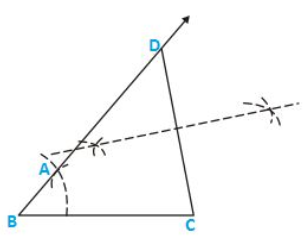Steps of Construction:

1. Construct a line segment BC of 3.6cm.
2. At the point B, draw ∠XBC=600.
3. Keeping B as center and radius 4.8cm draw an arc which intersects XB at D.
4. Join DC.
5. Draw the perpendicular bisector of DC which intersects DB at A.
6. Join AC.

Hence △ABC is the required triangle.

Q2. Construct a △ABC in which AB+AC=5.6cm ,BC=4.5cm and ∠B=450.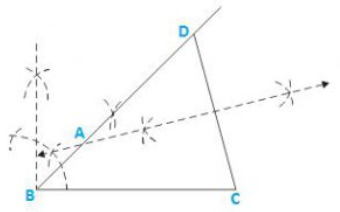Steps of Construction:

1. Construct a line segment BC of 4.5cm.
2. At the point B, draw ∠XBC=450.
3. Keeping B as centre and radius 5.6cm draw an arc which intersects XB at D.
4. Join DC.
5. Draw the perpendicular bisector of DC which intersects DB at A.
6. Join AC.

Hence △ABC is the required triangle

Q3. Construct a △ABC in which BC=3.4cm, AB-AC=1.5cm and ∠B=450.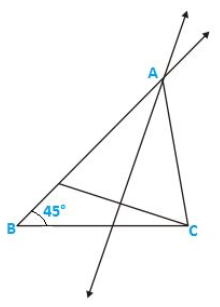Steps of Construction:

1. Construct a line segment BC of 3.4cm.
2. At the point B, draw ∠XBC=450.
3. Keeping B as centre and radius 1.5cm draw an arc which intersects XB at D.
4. Join DC.
5. Draw the perpendicular bisector of DC which intersects DB at A.
6. Join AC.

Hence △ABC is the required triangle.

Q4. Using rulers and compasses only, construct a △ABC, given base BC=7cm, ∠ABC=600 AB+AC=12cm.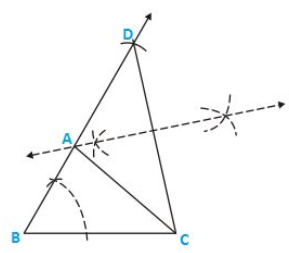Steps of Construction:

1. Construct a line segment BC of 7cm
2. At the point B, draw ∠XBC=600.
3. Keeping B as center and radius 12cm draw an arc which intersects XB at D
4. Join DC
5. Draw the perpendicular bisector of DC which intersects DB at A
6. Join AC

Hence △ABC is the required triangle.

Q5. Construct a triangle whose perimeter is 6.4cm, and angles at the base are 600 450.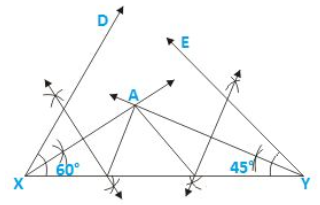Steps of Construction:

1. Draw a line segment XY of 6.4cm.
2. Draw ∠DXY=600 and ∠EYX=450.
3. Draw the angle bisectors of ∠DXY and ∠EYX which intersect each other at A.
4. Draw the perpendicular bisector of AX and AY which intersect XY at B and C respectively.
5. Join AB and AC.

Hence △ABC is the required triangle.

Q6. Using rulers and compasses only, construct a △ABC from the following data:

AB+BC+CA=12cm, ∠B=45and ∠C=600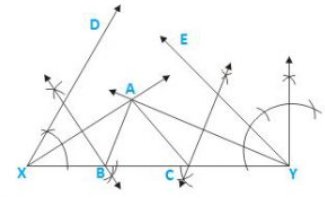Steps of Construction:

1. Draw a line segment XY of 12cm.
2. Draw ∠DXY=450 and ∠EYX=600.
3. Draw the angle bisectors of ∠DXY and ∠EYX which intersect each other at A.
4. Draw the perpendicular bisector of AX and AY which intersect XY at B and C respectively.
5. Join AB and AC.

Hence △ABC is the required triangle.

Q7. Construct a right-angled triangle whose perimeter is equal to 10cm and one acute angle equal to 600.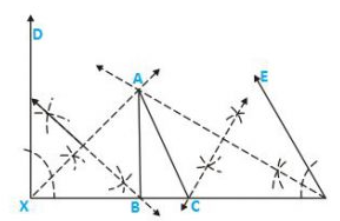Steps of Construction:

1. Draw a line segment XY of 10cm.
2. Draw ∠DXY=900 and ∠EYX=600.
3. Draw the angle bisectors of ∠DXY and ∠EYX which intersect each other at A.
4. Draw the perpendicular bisector of AX and AY which intersect XY at B and C respectively.
5. Join AB and AC.

Hence △ABC is the required triangle.

Q8. Construct a triangle ABC such that BC= 6cm, AB= 6cm and median AD=4cm.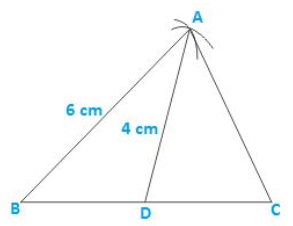Steps of construction:

1. Draw a line segment BC of 6cm.
2. Take mid-point O of side BC.
3. With center B and D and radii 6cm and 4cm, draw two arcs which intersect each other at A.
4. Join AB, AD and AC.

Hence △ABC is the required triangle.

Q9. Construct a right-angled triangle ABC whose base BC is 6cm and the sum of the hypotenuse AC and other side AB is 10cm.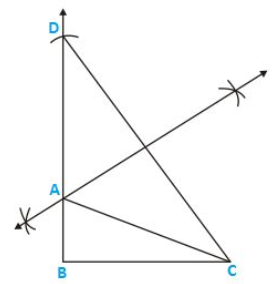Steps of Construction:

1. Construct a line segment BC of 6cm.
2. At the point B, draw ∠XBC=900.
3. Keeping B as center and radius 10cm draw an arc which intersects XB at D.
4. Join DC.
5. Draw the perpendicular bisector of DC which intersects DB at A.
6. Join AC.

Hence △ABC is the required triangle.

Q10. Construct a triangle XYZ in which ∠Y=300, ∠Z=900 and XY+YZ+ZX=11cm.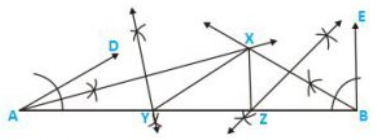Steps of construction:

1. Draw a line segment AB of 11cm.
2. Draw ∠DAB=300 and ∠FBA=900.
3. Draw the angle bisectors of ∠DAB and ∠EBA which intersect each other at X.
4. Draw the perpendicular bisector of XA and XB which intersect AB at Y and Z respectively
5. Join XY and XZ.

Hence △XYZ is the required triangle.

The document RD Sharma Solutions -Ex-17.3, Constructions, Class 9, Maths Notes | Study RD Sharma Solutions for Class 9 Mathematics - Class 9 is a part of the Class 9 Course RD Sharma Solutions for Class 9 Mathematics.
All you need of Class 9 at this link: Class 9Use Code STAYHOME200 and get INR 200 additional OFF Use Coupon Code

Top Courses for Class 9RD Sharma Solutions for Class 9 Mathematics

91 docs

Top Courses for Class 9Track your progress, build streaks, highlight & save important lessons and more!

,

,

,

,

,

,

,

,

,

,

,

,

,

,

,

,

,

,

,

,

,

,

,

,

,

,

,

,

,

,

;